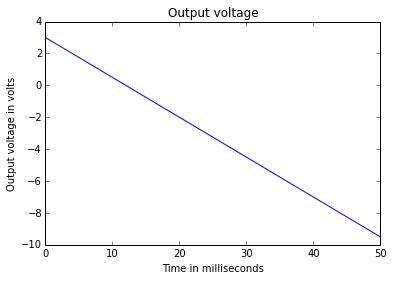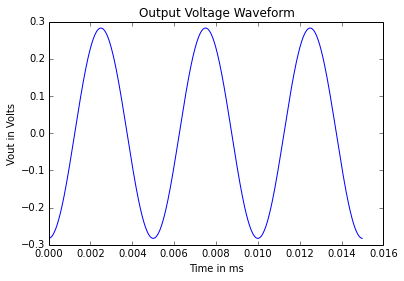# Chapter -4 Linear Applications of Op-Amps¶

## Example 4.1 - Page 111¶

In :
# Given data
Vout= '-(V1+10*V2+100*V3)' #given expression
Rf= 100 # in kΩ
# Vout= -Rf*(V1/R1+V2/R2+V3/R3)= -(Rf/R1*V1+Rf/R2*V2+Rf/R3*V3)     (i)
# Compare equation(i) with given expression
R1= Rf/1 #in kΩ
R2= Rf/10 # in kΩ
R3= Rf/100 # in kΩ
print "The value of Rf = %0.f kΩ" %Rf
print "The value of R1 = %0.f kΩ" %R1
print "The value of R2 = %0.f kΩ" %R2
print "The value of R3 = %0.f kΩ" %R3

The value of Rf = 100 kΩ
The value of R1 = 100 kΩ
The value of R2 = 10 kΩ
The value of R3 = 1 kΩ


## Example 4.2 - Page 112¶

In :
from __future__ import division
# Given data
Rf= 12 # in kΩ
R1= 12 # in kΩ
R2= 2 # in kΩ
R3= 3 # in kΩ
V1= 9 # in V
V2= -3 # in V
V3= -1 # in V
Vout= -Rf*(V1/R1+V2/R2+V3/R3) # output voltage in V
print "The output voltage = %0.f V" %Vout

The output voltage = 13 V


## Example 4.3 - Page 112¶

In :
# Given data
Vout= '(-V1+2*V2-3*V3)' #given expression
Rf= 6 # in kΩ
# Vout=  -(Rf/R1*V1+Rf/R2*V2+Rf/R3*V3)     (i)
# Compare equation(i) with given expression
R1= Rf/1 #in kΩ
R2= Rf/2 # in kΩ
R3= Rf/3 # in kΩ
print "The value of Rf = %0.f kΩ" %Rf
print "The value of R1 = %0.f kΩ" %R1
print "The value of R2 = %0.f kΩ" %R2
print "The value of R3 = %0.f kΩ" %R3

The value of Rf = 6 kΩ
The value of R1 = 6 kΩ
The value of R2 = 3 kΩ
The value of R3 = 2 kΩ


## Example 4.4 - Page 112¶

In :
# Given data
Vout= '(-2*V1+3*V2+4*V3)' #given expression
R3= 10 # in kΩ
# Vout=  -(Rf/R1*V1+Rf/R2*V2+Rf/R3*V3)     (i)
# Compare equation(i) with given expression
Rf= 4*R3 #in kΩ
R2= Rf/3 # in kΩ
R1= Rf/2 # in kΩ
print "The value of Rf = %0.f kΩ" %Rf
print "The value of R2 = %0.2f kΩ" %R2
print "The value of R1 = %0.f kΩ" %R1

The value of Rf = 40 kΩ
The value of R2 = 13.33 kΩ
The value of R1 = 20 kΩ


## Example 4.5 - Page 113¶

In :
V1= 2 # in V
V2= -1 # in V
R=100 # in Ω (assumed)
Vs1= V1*(R/2)/(R+R/2) # in V
Rf= 2*R # in Ω
Vo_1= Vs1*(1+Rf/R) # in V
Vs2= V2*(R/2)/(R+R/2) # in V
Vo_2= Vs2*(1+Rf/R) # in V
Vout= Vo_1+Vo_2 #output voltage in V
print "The output voltage = %0.f V" %Vout

The output voltage = 1 V


## Example 4.7 - Page 118¶

In :
# Given data
Vin= 10 # in V
R= 2.2 # in kΩ
R= R*10**3 # in kΩ
Ad= 10**5 # differential voltage gain
C=1 # in µF
C= C*10**-6 # in F
T= 1 # in ms
T= T*10**-3 # in s
I= Vin/R # in mA
V= I*T/C # output voltage at the end of pulse in mV
print "The output voltage at the end of the pulse = %0.3f V" %V
RC_desh= R*C*Ad # closed-loop time constant in sec.
print "The closed-loop time constant = %0.f seconds" %RC_desh

The output voltage at the end of the pulse = 4.545 V
The closed-loop time constant = 220 seconds


## Example 4.8 - Page 119¶

In :
# Given data
A_dB= 20 # peak gain in dB
A= 10**(A_dB/20) # peak gain
omega= 10000 # in rad/second
C= 0.01 # in µF
C= C*10**-6 # in F
Rf= 10 # in kΩ
# Vout/V1= Rf/R1= A
R1= Rf/A # in kΩ
print "The value of Rf = %0.f kΩ" %Rf
print "The value of R1 = %0.f kΩ" %R1

The value of Rf = 10 kΩ
The value of R1 = 1 kΩ


## Example 4.9 - Page 119¶

In :
%matplotlib inline
import numpy as np
from scipy.integrate import quad
from sympy import symbols
import matplotlib.pyplot as plt
from matplotlib.pylab import plot, show, ylim, xlim, text, subplot
a= symbols('a')
# Given data
R= 40 # in kΩ
R= R*10**3 # in Ω
C= 0.2 # in µF
C= C*10**-6 # in F
Vin= 5 # in V
V1= 3 # in V
t= 50 # in ms
Vout= 3 # in V
# Evaluation the integration
def integrand(x):
return (Vin-V1)
a=1
ans, err = quad(integrand, 0, 50)
# Output voltage when swith is open
vout= -1/(R*C)*ans*10**-3+Vout #in V
plt.plot([0,t],[Vout,vout])
plt.title('Output voltage')
plt.xlabel('Time in milliseconds')
plt.ylabel('Output voltage in volts')
plt.show()
print "Plot for output voltage shown in figure"Plot for output voltage shown in figure


## Example 4.10 - Page 120¶

In :
from scipy.integrate import quad
import numpy as np
# Given data
R= 500 # in kΩ
R= R*10**3 # in Ω
C= 10 # in µF
C= C*10**-6 # in F
vout= 12 # in V
v= -0.5 # in V
# given output equation : vout= -1/RC * integrate[v(t) * dt + A]
# Evaluation the integration
def integrand(t):
return -t
ans, err = quad(integrand, 0, 1)
vout_by_t= -1/(R*C)*ans #in V/sec
# Time required for saturation of output voltage
t= vout/vout_by_t # in sec
print "The time duration required for saturation of output voltage = %0.f seconds" %t

The time duration required for saturation of output voltage = 120 seconds


## Example 4.12 - Page 124¶

In :
from __future__ import division
%matplotlib inline
from sympy import symbols, simplify, sin
import matplotlib.pyplot as plt
import numpy as np
# Given data
fa= 1 # in kHz
fa=fa*10**3 # in Hz
Vp=1.5 # in volt
f= 200 # in Hz
C=0.1 # in micro F
C=C*10**-6 # in F
R= 1/(2*np.pi*fa*C) # in ohm
R=R*10**-3 # in k ohm
R=np.floor(R*10)/10 # in k ohm
fb= 20*fa # in Hz
R_desh= 1/(2*np.pi*fb*C) # in ohm
# Let
R_desh= 82 # in ohm
R_OM= R # in k ohm
print "Value of R_OM = %0.1f k ohm" %R_OM
CR= C*R
# Vin= Vp*sin(omega*t)= 1.5*sin(400*t)
# v_out= -CR*diff(v_in) = -0.2827 Cos(400*pi*t)# in micro volt
print "Output Voltage = -0.2827 Cos(400*pi*t)"
t = np.arange(0, .015, 1.0/44100)
v_out=-0.2827*np.sin(400*np.pi*t+np.pi/2)# in micro volt
plot(t,v_out)
plt.title('Output Voltage Waveform')
plt.xlabel('Time in ms')
plt.ylabel('Vout in Volts')
print "Output Voltage waveform is shown in figure."

Value of R_OM = 1.5 k ohm
Output Voltage = -0.2827 Cos(400*pi*t)
Output Voltage waveform is shown in figure.## Example 4.15 - Page 130¶

In :
# Given data
R1= 50 # in kΩ
R3=15 # in kΩ
R4=R3 # in kΩ
# For minimum differential voltage gain,
Ad_min= 5 # and
# From Ad= 1+2*R2/R1
R2= (Ad-1)*R1/2 # in kΩ
# For maximum differential voltage gain,
Ad_max= 200 # and

The value of R1 =  1 - 50 kΩ potentiometer